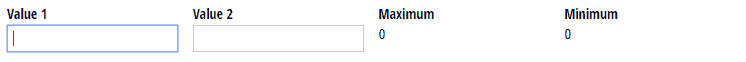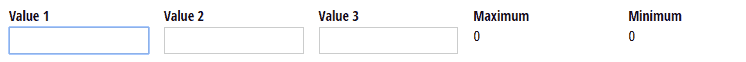# How can I find the highest or the lowest number out of multiple field values?

You can use the Maximum and Minimum functions in calculations to determine the highest or lowest number out of multiple field values.

To compare the minimum/maximum between two values:

1. Add two Number fields to your form (ex: “Value 1 and Value 2”).
2. Add a Calculation field to your form labelled ‘Minimum’ or ‘Maximum’. For your calculation, use the .Max or .Min function to compare the two fields: `=Math.Max(Value1,Value2)` or `=Math.Min(Value1,Value2)`
3. The Calculation field will now display either the highest or lowest value:To compare the minimum/maximum between more than two values:

1. Add three Number fields to your form (ex: “Value 1, Value 2, Value 3”).
2. Add a Calculation field to your form labelled ‘Minimum’ or ‘Maximum’. For your calculation, use the .Max or .Min function to compare the three fields: `=Math.Max(Value1, Math.Max(Value2,Value3))` or `=Math.Min(Value1, Math.Min(Value2,Value3))` Here, we’re setting up one function inside of another so that two of the values are compared first, and the resulting value is compared to the last one.
3. The Calculation field will now display either the highest or lowest value: